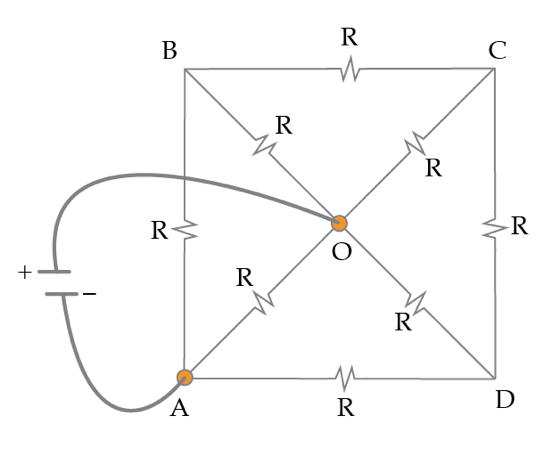# A resistors puzzle

In the figure below, all resistors have resistance $R=1~\Omega$. Find the equivalent resistance in Ohms between the points A and O.×